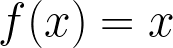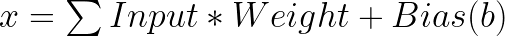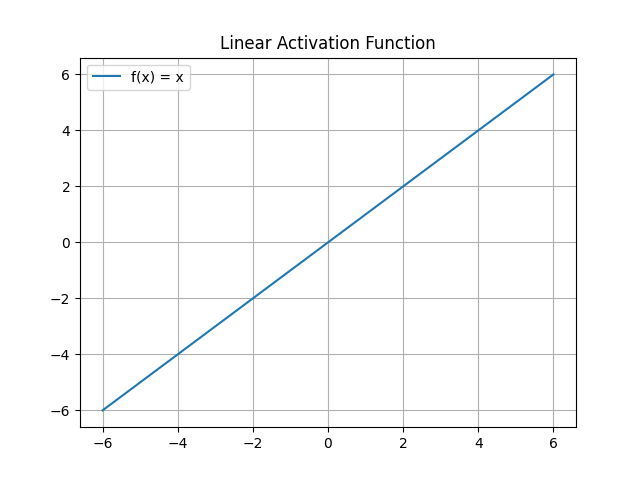# Linear Activation Function

The linear activation function, also known as the identity function, is one of the most straightforward activation functions, where the output is identical to the input. The linear activation function typically adds the weighted sum with bias and passes it as output.

Mathematically, it can be defined as:where :### Usage and Limitations

The problem with this activation function is that it is limited in the context of deep neural networks.

The model cannot learn complex non-linear relationships between inputs and outputs by using linear activation functions.

### Codebyte Example

The following is an example of the linear activation function in Python:

`Favicon IconCodeOutputLoading...`

This code will return a linear activation function graph: